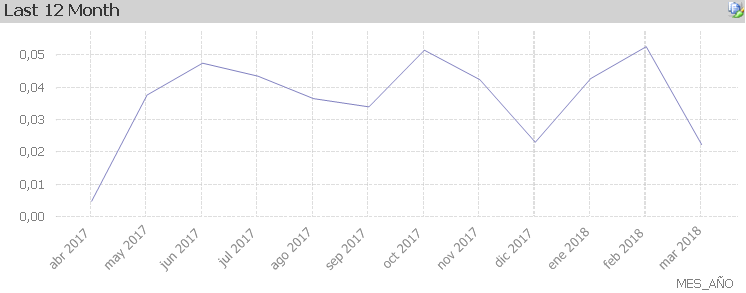# QlikView App Dev

Discussion Board for collaboration related to QlikView App Development.

Announcements
Action-Packed Learning Awaits! QlikWorld 2023. April 17 - 20 in Las Vegas: REGISTER NOW
cancel
Showing results for
Did you mean:Contributor II

## Expression to SUM two fields and DIVIDE by another field - NEED HELP

In my data table I have multiple columns. I want to create a column chart to display a rolling 12-month history of a certain metric. In order to calculate this metric, a sum of values between two of the columns from the data table must be added together conducive to the  filter selections, and then divided by a sum of values from a different column in the data table. Finally, multiply the result by one-million.

Example:

=SUM(SUM(COLUMN A),(COLUMN B) / SUM(COLUMN C))*1,000,000

Also, how do I get the months to show up on the X-Axis of the column chart? How do I make it dynamic to be a 12-month rolling?

Example:

...end-user selects [Year]=2019, rolling 12-mo chart should display current month at the end of X-axis, and the eleven months before it.

OR

...end-user selects [Year]=2019 AND [Month]=April, rolling 12-mo chart should display April 2019 at the end of the X-axis, and the eleven months into the prior year before it.

5 RepliesCreator III
hello, with the following expression you can get the last 12 months from the selection:
sum ({<Date = {'> = \$ (= AddMonths (Max (Date), - 12))'}>} Values)

Can you show a picture or upload a table with the data to see how to put together the expression?Contributor II
Author

I suspect I'll need to hard-code a new field in the load script. Instead of month's, like January, February, etc., I have fiscal periods. Example: '001' = April; '002' = May; etc...

The fiscal calendar starts in April and ends in March. I don't know how I'm going to hardcode this...Creator III

Use the next expression:

sum ({1<Date = {'> = \$ (= AddMonths (Max (Date), - 12))<\$ (= AddMonths (Max (Date), 0))'}>} Values)

that expression will show you in the graph the 12 months prior to the selected date, then if you select March 2018 it will show you all the values from March 2017Contributor II
Author

My data set contains a [FiscalYear] and [FiscalMoth] field.

Example:

[FiscalYear] = ('2017', '2018', '2019'); [FiscalPeriod] = ('001', '002', '003', '004', '005', '006', etc...)

The [FiscalPeriods] are actually MONTHS., however, January equals [FiscalPeriod] = '010'.

Our Fiscal Year begins in April, which is [FiscalPeriod] = '001'

I imagine I need to create some sort of cross-mapping table to help QlikView understand that, but I don't know where to start...Creator III

yes, you should create a link table in which you link the fiscal periods with the original calendar and where you should also format it so that the dates are recognized.